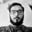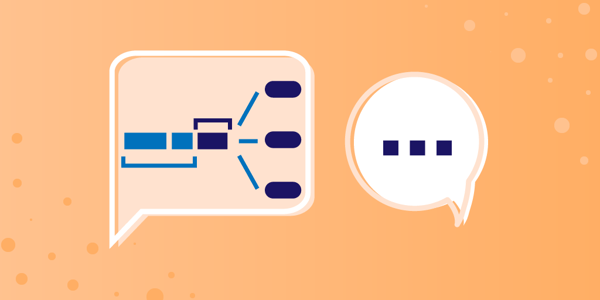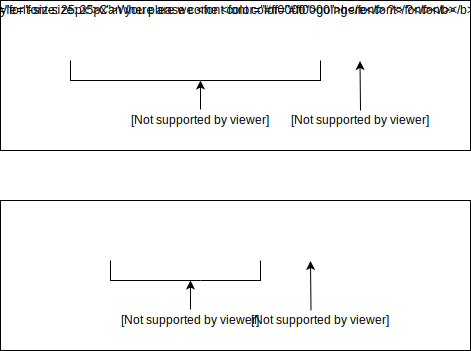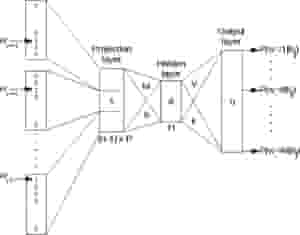# Data Science Simplified: What is language modeling for NLP?

Oct 14, 2020 - 9 min readAman AnandNatural Language Processing can perform a vast array of tasks, such as text summarization, generating completely new pieces of text, and word prediction, amongst others. All of these tasks are powered by language models.

Language models are an important component in any NLP journey. These language models power all the popular NLP applications: speech recognition, machine translation, part-of-speech tagging, parsing, and information retrieval and other applications.

Today, we will introduce language models, beginning basic models and then moving to State-of-the-Art language models that are trained using big data principles.

Today, we will cover:

## What is language modeling?

Language modeling (LM) analyzes bodies to text to provide a foundation for word prediction. These models use statistical and probabilistic techniques to determine the probability of a particular word sequence occurring in a sentence.

Language modeling is used in NLP techniques that generate written text as an output.

Applications and programs with NLP-concepts rely on language models for tasks like audio-to-text conversion, sentiment analysis, speech recognition, and spelling corrections.

Language models work by determining word probabilities in an analyzed chunk of text data. Data is interpreted after being fed to a machine learning algorithm that looks for contextual rules in that given natural language (i.e. English, Japanese, Spanish).

The model then applies those rules to the input language tasks for generating predictions. It can even produce new sequences or sentences based on what it learned.Language models are useful for both text classification and text generation. In text classification, we can use the language model’s probability calculations to separate texts into different categories.

For example, if we trained a language model on spam email subject titles, the model would likely give the subject “CLICK HERE FOR FREE EASY MONEY” a relatively high probability of being spam.

In text generation, a language model completes a sentence by generating text based on the incomplete input sentence. This is the idea behind the autocomplete feature when texting on a phone or typing in a search engine. The model will give suggestions to complete the sentence based on the words it predicts with the highest probabilities.

## Types of Language Models

There are two categories that Language Models fall under:

Statistical Language Models: These models use traditional statistical techniques like N-grams, Hidden Markov Models (HMM), and established linguistic rules to learn the probability distribution of words. Statistical Language Modeling involves the development of probabilistic models that can predict the next word in the sequence given the words that precede it.

Neural Language Models: These models are new players in the NLP world and have surpassed the statistical language models in their effectiveness. They use different kinds of Neural Networks to model language. The use of neural networks in the development of language models has become so popular that it is now the preferred approach for challenging tasks like speech recognition and machine translation.

Note: GPT-3 is an example of a Neural language model. BERT by Google is another popular Neural language model used in the algorithm of the search engine for next word prediction of our search query.

## Introduction to Statistical language models

Let’s take a deeper dive into the concept of Statistical language models. A language model learns the probability of word occurrence based on examples of text. Simpler models may look at a context of a short sequence of words, whereas larger models may work at the level of sentences or paragraphs. Most commonly, language models operate at the level of words.### N-gram Language Models

The n-gram model is a probabilistic language model that can predict the next item in a sequence using the (n − 1)–order Markov model. Let’s understand that better with an example. Consider the following sentence:

#### “I love reading blogs on Educative to learn new concepts”

A 1-gram (or unigram) is a one-word sequence. For the above sentence, the unigrams would simply be: “I”, “love”, “reading”, “blogs”, “on”, “Educative”, “and”, “learn”, “new”, “concepts”.

A 2-gram (or bigram) is a two-word sequence of words, like “I love”, “love reading”, “on Educative” or “new concepts”.

Lastly, a 3-gram (or trigram) is a three-word sequence of words, like “I love reading”, “blogs on Educative”, or “learn new concepts”.

An N-gram language model predicts the probability of a given N-gram within any sequence of words in the language. If we have a good N-gram model, we can predict $p(w | h)$, or the probability of seeing the word w given a history of previous words h, where the history contains n-1 words.

Example: “I love reading ___”. Here, we want to predict what word will fill the dash based on the probabilities of the previous words.

We must estimate this probability to construct an N-gram model. We compute this probability in two steps:

1. Apply the chain rule of probability
2. We then apply a very strong simplification assumption to allow us to compute p(w1…ws) in an easy manner.

The chain rule of probability is:

$p(w1...ws) = p(w1) . p(w2 | w1) . p(w3 | w1 w2) . p(w4 | w1 w2 w3) ..... p(wn | w1...wn-1)$

Definition: What is the chain rule? It tells us how to compute the joint probability of a sequence by using the conditional probability of a word given previous words.

Here, we do not have access to these conditional probabilities with complex conditions of up to n-1 words. So, how do we proceed? This is where we introduce a simplification assumption. We can assume for all conditions, that:

$p(wk | w1...wk-1) = p(wk | wk-1)$

Here, we approximate the history (the context) of the word wk by looking only at the last word of the context. This assumption is called the Markov assumption. It is an example of the Bigram model. The same concept can be enhanced further for example for trigram model the formula will be:

$p(wk | w1...wk-1) = p(wk |wk-2 wk-1)$

These models have a basic problem: they give the probability to zero if an unknown word is seen, so the concept of smoothing is used. In smoothing we assign some probability to the unseen words. There are different types of smoothing techniques such as Laplace smoothing, Good Turing, and Kneser-ney smoothing.

## Introduction to Neural language models

Neural language models have some advantages over probabilistic models. For example, they don’t need smoothing, they can handle much longer histories, and they can generalize over contexts of similar words.

For a training set of a given size, a neural language model has much higher predictive accuracy than an n-gram language model.

On the other hand, there is a cost for this improved performance: neural net language models are strikingly slower to train than traditional language models, and so for many tasks an N-gram language model is still the right tool.

In neural language models, the prior context is represented by embeddings of the previous words. This allows neural language models to generalize unseen data much better than N-gram language models.From Semantic Scholar

Word embeddings are a type of word representation that allow words with similar meaning to have a similar representation. Word embeddings are, in fact, a class of techniques where individual words are represented as real-valued vectors in a predefined vector space.

Each word is mapped to one vector, and the vector values are learned in a way that resembles a neural network. Each word is represented by a real-valued vector, often tens or hundreds of dimensions.

Note: Some of the word embedding techniques are Word2Vec and GloVe.

The Neural language models were first based on RNNs and word embeddings. Then the concept of LSTMs, GRUs and Encoder-Decoder came along. The recent advancement is the discovery of Transformers, which has changed the field of Language Modelling drastically.

Some of the most famous language models like BERT, ERNIE, GPT-2 and GPT-3, RoBERTa are based on Transformers.

The RNNs were then stacked and used bidirectionally, but they were unable to capture long term dependencies. LSTMs and GRUs were introduced to counter this drawback.

The transformers form the basic building blocks of the new neural language models. The concept of transfer learning is introduced which was a major breakthrough. The models were pre-trained using large datasets.

For example, BERT is trained on the entire English Wikipedia. Unsupervised learning was used for training of the models. GPT-2 is trained on a set of 8 million web pages. These models are then fine-tuned to perform different NLP tasks.

## What to learn next

Well done! You should now have a good understanding of language models of NLP. This knowledge will be instrumental in your machine learning journey as you learn how to implement these models yourself.

There is still a lot to learn about Natural Language Processing. I recommend learning the following concepts next to expand your knowledge:

• TensorFlow with Python
• Skip-gram for word embeddings
• Tensor indexing
• Text classification
• Seq2Seq Models

To get started with these topics, check out Educative’s course Natural Language Processing with Machine Learning. In this course, you’ll learn techniques for processing text data, creating word embeddings, and using long short-term memory networks (LSTM) for tasks such as semantic analysis and machine translation. Knowledge of Python and TensorFlow are prerequisites.

Happy learning!